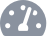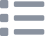# Line Tracking Module with Robit Smart Car

## Get the Robit Smart Car to detect edges with the tracking module

Written By: Cherie TanDifficulty
EasySteps
9

### Introduction

Wouldn't it be cool to have the Robit Smart Car move around on a surface above ground, without it falling off?

In this guide, you will learn to connect and use the line tracking module to the Robit Smart Car. It will be programmed in MakeCode to perform edge detection.

Complete this guide to have a Robit Smart Car that can detect edges above ground.

### Step 1   Connect module and motors to Robit motherboard• In this guide, you will learn to use the line tracking module. This module has an infrared sensor which consists of a transmitter and a receiver. While the transmitter emits infrared light, the receiver can detect the infrared light reflected by the ground.
• When running on black-coloured ground or objects, which absorb infrared light, the receiver will not be able to detect infrared light.
• If you haven't done so already, head over to the Robit Smart Car product page for instructions on how to assemble the smart car. For this guide, we will need:

The line tracking module is to be connected to the robit motherboard as shown in the first image. Make sure two 3-pin wires are connected to Pin 15 and Pin 16 on the motherboard.

The two motors are to be connected to M1 and M2.

Connect the other end of the 3-pin wires to line detection module as shown in the third image

### Step 2   Install MakeCode package• First, open MakeCode Editor
• Click on 'New Project'
• To easily program the Robit Smart Car, we'll use the 'robit' MakeCode package over in the Extensions tab. Click on 'Advanced'
• Click on 'Extensions'
• Type 'robit' in the search bar
• Click on the robit package and it will be automatically added to the MakeCode editor

### Step 3   On start

```robit.init_line_follow(robit.Jpin.J2)
robit.MotorRunDual(
robit.Motors.M1,
20,
robit.Motors.M2,
20
)
```
• Copy and paste the following code into the Javascript interface.

### Step 4   Create variables

```let right = 0
let left = 0
robit.init_line_follow(robit.Jpin.J2)
robit.MotorRunDual(
robit.Motors.M1,
20,
robit.Motors.M2,
20
)
basic.forever(function () {
left = 0
right = 0
})
```
• Replace the code with the following.
• Two variables have been created, 'left' and 'right'.

### Step 5   Set variables to '... line follow digitalpin' blocks

```let right = 0
let left = 0
robit.init_line_follow(robit.Jpin.J2)
robit.MotorRunDual(
robit.Motors.M1,
20,
robit.Motors.M2,
20
)
basic.forever(function () {
left = robit.left_line_follow()
right = robit.right_line_follow()
})
```
• Replace the existing code with the following in the Javascript interface.

### Step 6   Set a condition

```let right = 0
let left = 0
robit.init_line_follow(robit.Jpin.J2)
robit.MotorRunDual(
robit.Motors.M1,
20,
robit.Motors.M2,
20
)
basic.forever(function () {
left = robit.left_line_follow()
right = robit.right_line_follow()
if (!(right || left)) {
robit.MotorRunDual(
robit.Motors.M1,
-20,
robit.Motors.M2,
-20
)
basic.pause(500)
} else {

}
})
```
• Replace the existing code with the following in the Javascript interface.
• What this means is if the right and left variables are '0' then do the following (within the if block). So, if the right or left variables are '0', this means the tracking module's infrared sensor has detected edges. To prevent the Robit Smart Car from falling off the edge, we'll set the speed of the motors to be negative; this causes it to reverse.

### Step 7   Add an if block inside the if block

```let num = 0
let right = 0
let left = 0
robit.init_line_follow(robit.Jpin.J2)
robit.MotorRunDual(
robit.Motors.M1,
20,
robit.Motors.M2,
20
)
basic.forever(function () {
left = robit.left_line_follow()
right = robit.right_line_follow()
if (!(right || left)) {
robit.MotorRunDual(
robit.Motors.M1,
-20,
robit.Motors.M2,
-20
)
basic.pause(500)
num = Math.randomRange(0, 100)
if (num < 50) {
robit.MotorStop(robit.Motors.M1)
basic.pause(300)
} else if (num > 50) {
robit.MotorStop(robit.Motors.M2)
basic.pause(300)
} else {

}
} else {

}
})
```
• Replace the existing code with the following.

### Step 8   Complete MakeCode

```let num = 0
let right = 0
let left = 0
robit.init_line_follow(robit.Jpin.J2)
robit.MotorRunDual(
robit.Motors.M1,
2,
robit.Motors.M2,
15
)
basic.forever(function () {
left = robit.left_line_follow()
right = robit.right_line_follow()
if (!(right || left)) {
robit.MotorRunDual(
robit.Motors.M1,
-20,
robit.Motors.M2,
-20
)
basic.pause(500)
num = Math.randomRange(0, 100)
if (num < 50) {
robit.MotorStop(robit.Motors.M1)
basic.pause(300)
} else if (num > 50) {
robit.MotorStop(robit.Motors.M2)
basic.pause(300)
}
} else {
robit.MotorRunDual(
robit.Motors.M1,
20,
robit.Motors.M2,
20
)
}
})
```
• Now that we've programmed the conditions for when an edge is detected, what about if the infrared sensor does not detect an edge? To complete the MakeCode, we'll focus on the 'else' condition. Replace the code with the following in the Javascript interface.
• If the infrared sensor in the tracking module does not detect an edge, motor speed is set to 20. This means the smart car will continue to move forward.

### Step 9   Upload to micro:bit• To upload the code to the micro:bit, first connect the micro:bit to the computer via microUSB cable